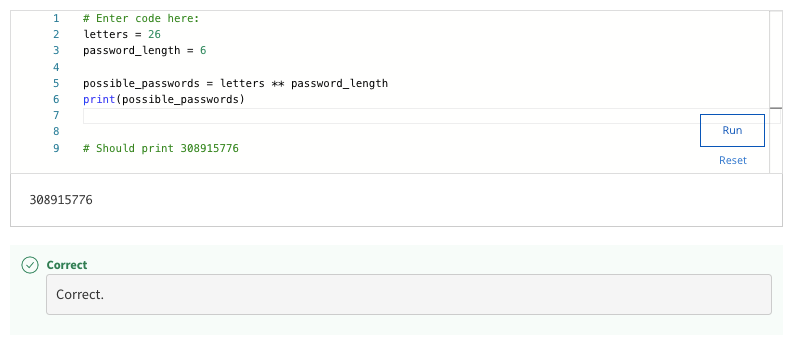## crash course on python week 1 quiz answers – Shuffle Q/A 1

• Syntax
• Grammar
• Format
• Semantics

#### 29. Complete the code so that the string "I am writing Python code!" will print to the screen. Remember that syntax precision is important in programming languages. A missing capital letter, spelling error, or punctuation mark can produce errors.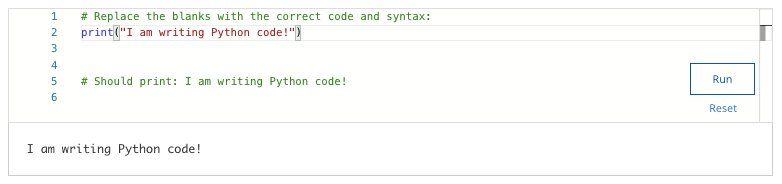• 49.0
• 0.28
• 20.0
• 19.36

#### 31. Assuming there are 60 minutes in an hour, write a program that calculates the number of minutes in a 24 hour day. Print the result on the screen. Note: Your result should be in the format of just a number, not a sentence.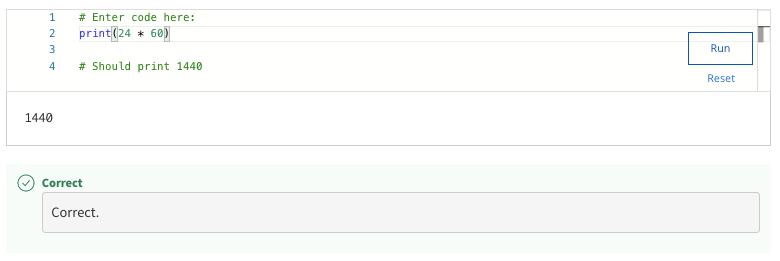#### 32. Mercury has a diameter of approximately 1,516 miles. Earth has a diameter of approximately 3,959 miles. Use Python to calculate how much larger Earth’s diameter is than Mercury's (in miles). Note: Your result should be in the format of a number, not a sentence.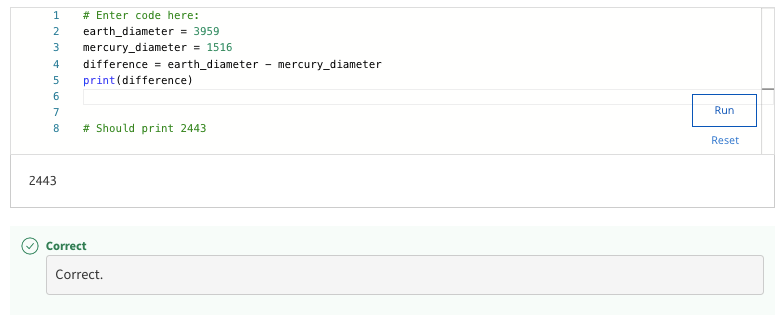#### 33. Consider this scenario about using Python to make calculations:On a college campus, there are 30 computers in each of the 20 computer labs that are spread across campus. The computers have a life cycle where they are replaced every 5 years, with an equal number (one-fifth) of the computers replaced each year. Fill in the blank to compute the number of computers that are replaced each year. Note: Your result should be in the format of just a number, not a sentence.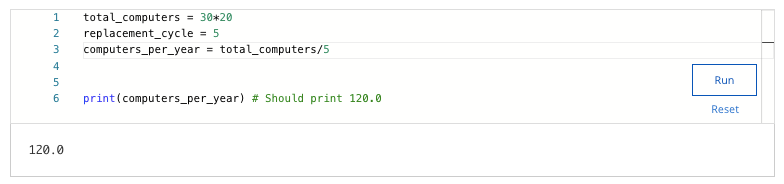#### 34. What is a computer program?

• A file that gets printed by the Python interpreter.
• The syntax and semantics of a programming language.
• The overview of what the computer will have to do to solve an automation problem.
• Step-by-step instructions on how to complete a set of tasks, to be executed by a computer.

#### 35. Which of the following are characteristics of the Python language? Select all that apply.

• Python is cross-platform compatible
• Python is used in a wide variety of applications
• Python has many platform-specific tools, like Bash or Powershell
• Python is an object-oriented language not fit for general purpose scripting

#### 36. Keeping in mind there are 86400 seconds per day, write a program that calculates how many seconds there are in a week, if a week is 7 days. Print the result to the screen. Note: Your result should be in the format of just a number, not a sentence.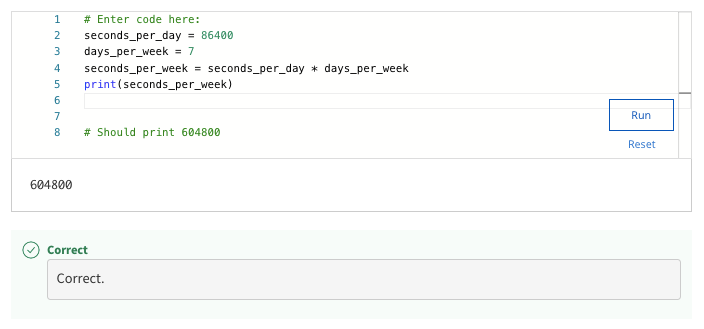#### 37. Use Python to calculate how many number-based passcodes can be formed with 10 numerals (0 through 9). For a 1 numeral passcode, there would be 10 possibilities. For a 2 numeral passcode, each numeral is independent of the other, so there would be 10 times 10 possibilities. Using this information, print the amount of possible passwords that can be formed with 8 numerals. Note: Your result should be in the format of just a number, not a sentence.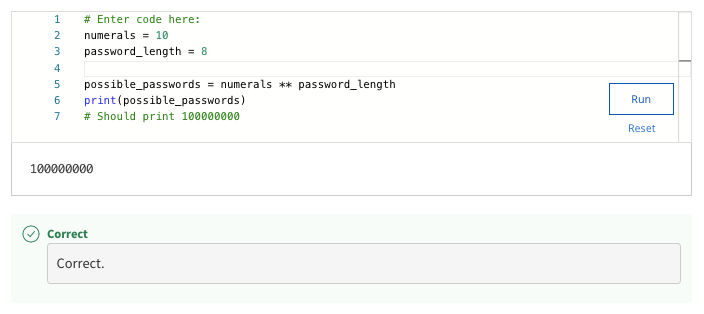# Shuffle Q/A 2

#### 38. What is the difference between syntax and semantics in a programming language?

• Syntax is a set of rules for how statements are constructed. Semantics refers to the intended meaning or effect of statements.
• Syntax is the effect the instructions have on the system and semantics are how to write the instructions.
• Syntax is the tool that executes a computer program and semantics is the development environment.
• Syntax refers to computer programs and semantics is another word for scripts.

#### 39. Use Python to calculate how many different passwords can be formed with 6 lower case English letters (excludes any character not found in the English alphabet). For a 1 letter password, there would be 26 possibilities. For a 2 letter password, each letter is independent of the other, so there would be 26 times 26 possibilities. Using this information, print the amount of possible passwords that can be formed with 6 letters.